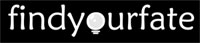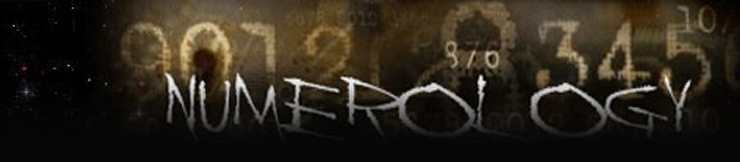# Hebrew Numerology

Gematria is an age old Jewish phenomenon in which the numerical values of letters, words, or phrases are calculated and then based on it different concepts are analyzed and explored thus leading to association between the words and its ideas. Apparently being derived from a Greek word but still it is most used in Jewish texts particularly associated with Kabbalah.

In this when numerical values are assigned to each letter or word there said to exist some relation to the number and the word which is then rendered with the person's age, calendar year and other factors. The Mispar gadol table followed by the Jewish people assigns the number 18 after some particular calculation to the word Chai which means life. Hence this number 18 is considered very lucky among the Jews.The calculation of the numerical equivalent value of a word in Greek is known by the term Isopsephy.Though the English words doesn't carry any numerical value but soon it started following the numbering system and after which referred by the term English Gematria. The hypothesis behind Gematria the numerical equality between words and the numbers is not something which is a mere coincidence but it is believed that each letter have a different meaning with a creative force from the delivery of God's speech.

There are two forms of Gematria one is the revealed form and the other one is the mystical form. Gematria is said to be dated back to Tannaic period and this information is sourced from a Mishnaic text. The numerical value associated with each letter can be in Gematria by four basic methods, and they are

Absolute Value

Ordinal Value

Reduced Value

Integral Reduced value

There is a text source from Tikunei Zohar which says that the intention or the idea behind the reduced value is that it is associated with the spiritual world of Yetzirah which arrives at a connection between these different forms of calculation including the four letters of God's name, and the four religious kingdoms.

Letter of God's essential
Name, Havayah

Type of calculation

World

yud

absolute value

emanation (atzilut)

hei

ordinal value

creation (beri'ah)

vav

reduced value

formation (yetzirah)

hei

integral reduced value

action (asiyah)

Below is the significance for some individual numbers based on the number theory and its relation with the words and phrases which is equivalent to that particular number. These words and phrases are mainly sourced from the text of Torah literature.

Number 0 - According to Kabbalah zero is not the same as the other numbers and it is revealed in its concealed form.

Number 1378 - According to the number theory this denotes the 52nd triangular number.

Number 1513 - According to the number theory this denotes the 28th inspirational number.

Number 1839 - According to Chassidut 1839 is the value of the three words "Torah", "prayer", "repentance".

Number 306 - According to the number theory 306 is the 17th diamond number.

Number 358 - According to Chassidut 358 is the gematria of "emissary" and the 10 powers of the soul.

Number 377 - According to the number theory 377 is the 14th love number.

Number 543 - In gematria it is the numerical value for the words "truth" and "faith".

Number 66 - This is the 11th triangular number according to the number theory.

Number 685 - This is the 19th inspirational number according to the number theory.

Number 819 - In gematria it is the numerical value for the word simple unity.

## Gematria Methods

### Absolute Value

This is also referred by the name Normative value. In this method of calculation each letter is provided with its numerical equivalent, say the first letter equals 1, the second letter equals 2 and it goes like that where the tenth letter is deemed to the numeral 10 and from this successive number it is equivalent to 20, 30, and 40 and so on. Towards the end and not the last takes up the numerical equivalent 100 and finally the last letter equals to 400.The calculation of the final forms of the letters which are used to conclude a word like chaf, mem, nun, pei, and tzadik are generally assigned the numerical equivalent of the standard form of the letter. When the calculation of the alternate form is considered then it is a complete cycle for the Hebrew alphabet where the final tzadik equals 900 and alef is equivalent to the numerical one and one thousand.

### Ordinal Value

According to the ordinal value calculation in the gematria method each of the 22 letters are assigned to its numerical equivalent say from 1 to 22 with

alef = 1

Kaf = 11

taf = 22

final kaf = 23

### Reduced value

According to the reduced value calculation in Gematria the letters doesn't have 22 equivalents but only 9 where

alef = 1

yud = 10

kuf = 100

### Integral Reduced Value

In this method of computing a number, it is based on the arrival of a single digit integer where the total numerical value of a word is added repeatedly again and again till it ends up with a single digit number which is regardless of absolute, ordinal or the reduced values.

Let us now see one simple calculation by the Gematria method. The English word loving-kindness is pronounced as chesed in Hebrew which is spelt as chet, samech, dalet.Thus it has 3 letters. According to the calculation by the absolute value method chet is assigned the numerical value 8,samech takes the value 60 and the last dalet is equivalent to 4.Now when you add up all these numbers you arrive at an answer of 72,which when further reduced will end up with 9.This is the integral reduced value of the word chesed.Now when we consider the calculation by the ordinal value method chet is assigned 8,dalet takes up the numeral 4 and samech here is equivalent to the number 15.All these three when added up gives you a value of 27 which when further reduced gives you an integral reduced value of 9.The reduced value of calculation assigns the number 8 to chet,6 to samech and 4 to dalet which when added ends up in a sum of 18 and once again the integral reduced value of this number is 9.

### Filling of Letters

Sometime in the Gematria method the numerical value calculation of a word or a letter is also done by another way which is known by the name milui meaning filling. It is just not a mathematical way of calculation but it is believed that a spiritual or a heavenly influence is associated with each numerical equivalent. In this method of letter filling apart from each letter taking up a numerical equivalent it is also regarded as a complete word. Let us see an example, when word is made of chet and nun. Here chet and nun are respectively inturn spelt as

Chet, yud, tav = 418

nun, vav, nun = 106

Now the value of chen by the letter filling method is 524.

Let us consider the divine name Havayah which is usually spelt as yud, hei, vav, hei by the letter filling method whose numerical value by the gematria method is 26.This keeps varying by the letter filling method when it is spelled in a different way. It is usually said that they have a three different spellings which is supposed to have at least 13 numerical equivalent which ranges between 44 to 72.This after the calculation by the miluim method equals to 39 which means Hashem Echad ("God is One").So the association between the words and the numbers which arrives at a conclusion is most of the time uncertain and it depends on each person's belief and prejudice view. Thus in such cases arises the need for the calculation of the numerical equivalents of the words like Gematria.